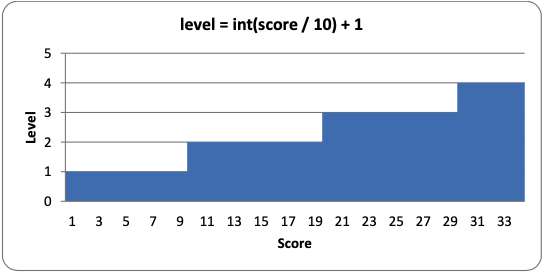# 8.25: The calculateLevelAndFallFreq() Function

def calculateLevelAndFallFreq(score):
# Based on the score, return the level the player is on and
# how many seconds pass until a falling piece falls one space.
level = int(score / 10) + 1
fallFreq = 0.27 - (level * 0.02)
return level, fallFreq


Every time the player completes a line, their score will increase by one point. Every ten points, the game goes up a level and the pieces start falling down faster. Both the level and the falling frequency can be calculated from the score that is passed to this function.

To calculate the level, we use the int() function to round down the score divided by 10. So if the score any number between 0 and 9, the int() call will round it down to 0. The + 1 part of the code is there because we want the first level to be level 1, not level 0. When the score reaches 10, then int(10 / 10) will evaluate to 1, and the + 1 will make the level 2. Here is a graph showing the values of level for the scores 1 to 34:To calculate the falling frequency, we start with a base time of 0.27 (meaning that the piece will naturally fall once every 0.27 seconds). Then we multiply the level by 0.02, and subtract that from the 0.27 base time. So on level 1, we subtract 0.02 * 1 (that is, 0.02) from 0.27 to get 0.25. On level 2, we subtract 0.02 * 2 (that is, 0.04) to get 0.23. You can think of the level * 0.02 part of the equation as "for every level, the piece will fall 0.02 seconds faster than the previous level."

We can also make a graph showing how fast the pieces will fall at each level of the game:You can see that at level 14, the falling frequency will be less than 0. This won’t cause any bugs with our code, because line 277 just checks that the elapsed time since the falling piece last fell one space is greater than the calculated falling frequency. So if the falling frequency is negative, then the condition on line 277 will always be True and the piece will fall on every iteration of the game loop. From level 14 and beyond, the piece cannot fall any faster.

If the FPS is set at 25, this means that at reaching level 14, the falling piece will fall 25 spaces a second. Considering that the board is only 20 spaces tall, that means the player will have less than a second to set each piece!

If you want the pieces to start (if you can see what I mean) falling faster at a slower rate, you can change the equation that the calculateLevelAndFallFreq() uses. For example, let’s say line 5  was this:

fallFreq = 0.27 - (level * 0.01)


In the above case, the pieces would only fall 0.01 seconds faster on each level rather than 0.02 seconds faster. The graph would look like this (the original line is also in the graph in light grey):As you can see, with this new equation, level 14 would only be as hard as the original level 7. You can change the game to be as difficult or easy as you like by changing the equations in calculateLevelAndFallFreq().